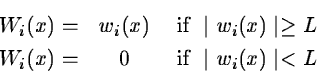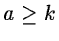Next: Comparison using a multiresolution Up: Noise reduction from the Previous: Adaptive filtering from the

In the previous algorithm we do not use the hierarchy of structures. We have explored many approaches for introducing a non-linear hierarchical law in the adaptive filtering and we found that the best way was to link the threshold to the wavelet coefficient of the previous plane wh. We get:and L is a threshold estimated by:where Sh is the standard deviation of wh. The function t(a)must return a value between 0 and 1. A possible function for t is:
• t(a) = 0 if•if a < k

Petra Nass
1999-06-15